# Lecture Seven

GET STARTED
1
Request Info
2
Visit
3
Apply
GET STARTED
1
Request Info
2
Visit
3
Apply

## Lectures 7 & 8Mass, salt, heat, and momentum conservation and the Coriolis effect

The way we understand the ocean is based on the simple concept of CONSERVATION. If we consider an arbitrary volume of water, a “parcel”, then over that volume mass must be conserved (it cannot be created or destroyed). Temperature and salinity can only change as a result of the flow of warmer/colder water or saltier/fresher waters across the boundaries of the volume, or due to the diffusion of temperature or salt into the volume.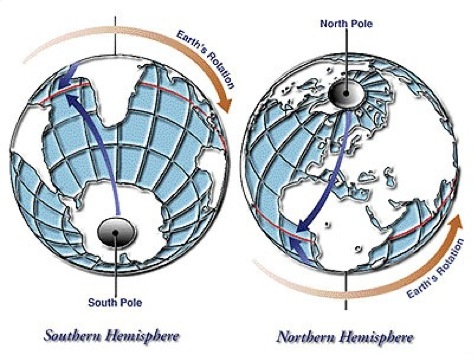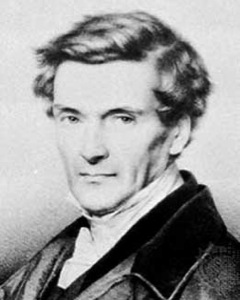Notes

##### 1. Mass Conservation Equation and Continuity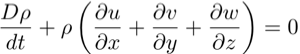We note that the first term, the change in density with time (moving with a fluid parcel) is very small for time scales larger than a few seconds. In other words, except for sound waves, the compressibility of the water parcel is unimportant and can be neglected. Hence we find the CONTINUITY EQUATION, which says that the divergence of the flow is zero - that is, the flow is NON-DIVERGENT.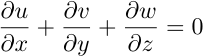##### 2. Salt Conservation

The equation for salt conservation can be developed similarly to that for mass, but now we need to consider exchanges of salt via diffusive fluxes. Diffusion acts on the gradient of salinity.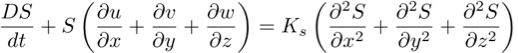where K_s is the diffusion coefficient of salt. This is simplified when we consider a non-divergent flow: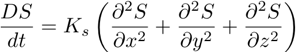##### 3. Heat Conservation

Energy has several forms in the ocean: kinetic and potential energies (mechanical), internal energy, and chemical energy. We can measure internal energy by measuring the temperature of the fluid. Mechanical and chemical energy dissipation into heat is small. By far the largest contribution to heat in the ocean comes from solar radiation and the surface heat flux. Again, we assume non-divergent flow.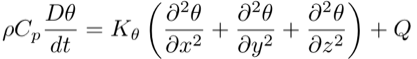where Cp is the heat capacity of sea water and K_theta is the diffusion coefficient for temperature (100x larger than K_s). Q is the heating due to mechanical or chemical energy dissipation (small), or due to heat exchange with the atmosphere.

##### 4. Momentum Conservation and the Coriolis force

For the conservation of momentum we must consider the advection of momentum across the walls of our fluid parcel plus all external forces on the parcel that may change its momentum, in other words, all forces that can accelerate the flow. The external forces are pressure gradients, gravity, and shear stresses (in the form of eddy viscosity). We must also consider the affect of ROTATION on the flow. In transforming from an inertial (non-accelerating) frame to a rotating (accelerating) frame, such as we live in here on earth, we derive the CORIOLIS FORCE. For ocean circulation the time and space scales of the flow are such that the Coriolis effect is important.

There are three momentum equations, one each for the u, v, and w components of the flow.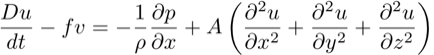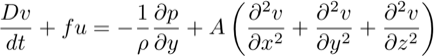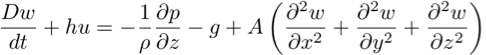where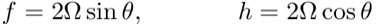and A is the kinematic eddy viscosity (coefficient of viscosity divided by density)

##### 4. Inertial Oscillations

Inertial oscillations are motions in the ocean that occur in response to an impulse force. Such a force could be a wind event, for example, that sets the water in motion and then leaves it to “restore” under the influence of only the Coriolis force. With no external forces, the horizontal Equations of Motion become: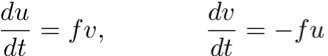These equations are coupled, first order, linear differential equations and can be solved. If we re-arrange the second equation and substitute it into the first we should recognise the equation for a harmonic oscillator.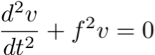with solution,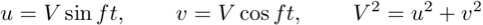these equations describe a circle of radius R = V/f and period, T = 2∏/f.
Inertial currents are the most common motions in the ocean. They are only a few kilometers in scale and decay in a few days.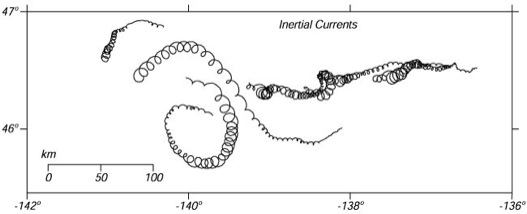Inertial currents in the North Pacific in October 1987 (days 275-300) measured by holey-sock drifting buoys drogued at a depth of 15 meters. Positions were observed 10-12 times per day by the Argos system on NOAA polar-orbiting weather satellites and interpolated to positions every three hours. The largest currents were generated by a storm on day 277. Note: these are not individual eddies. The entire surface is rotating. A drogue placed anywhere in the region would have the same circular motion. From van Meurs (1998).

##### Extra material:

Movie illustrating the Coriolis effect:
http://www.classzone.com/books/earth_science/terc/content/visualizations/es1905/es1905page01.cfm

An in-depth look at the Coriolis effect by Jim Price at MIT/WHOI:
http://ocw.mit.edu/ans7870/resources/price/index.htm